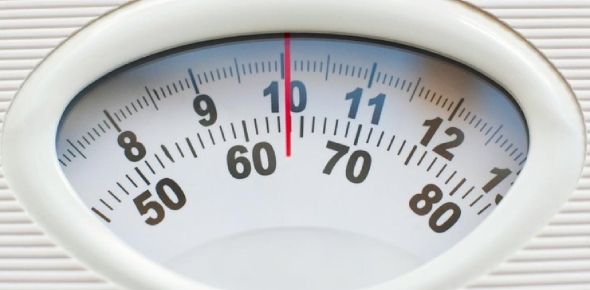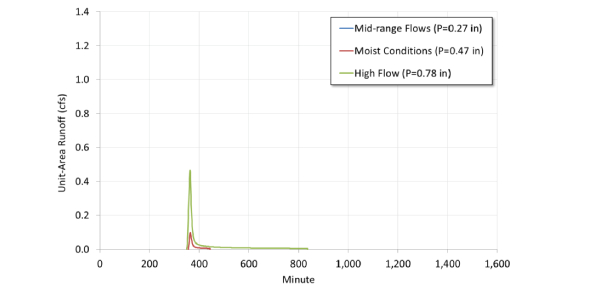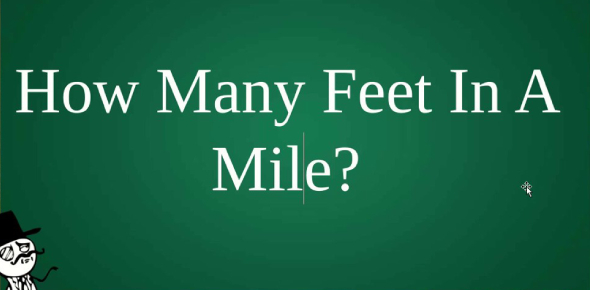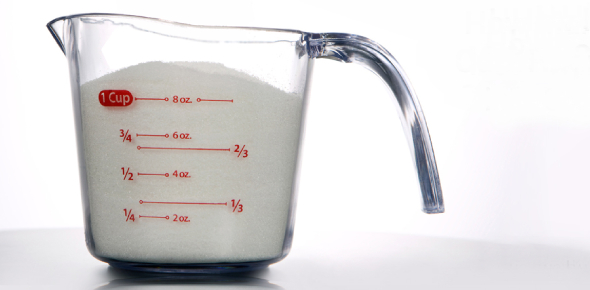# Si Units Questions and Answers (Q&A)#### Kendrell

Gallons to cups conversion factors are listed below (for US, UK and for both fluid and dry measurements). To find out how many cups in a gallon, multiply by the conversion factor or use the converter if the conversion is from US fluid gallons to US cups. 1 Gallon [Fluid, US] = 16 Cups [US], 1 Gallon [Dry, US] = 18.618355 Cups [US], 1 Gallon [UK] = 18.18436 Cups [Metric] There are 16 cups in a US fluid gallon, because from the largest to the smallest units from gallon to cup, there are 4 quarts in a gallon, 2 pints in a quart and 2 cups in each pint, that makes 4 2 2 = 16 cups in a gallon.

The imperial and the Canadian cups are no longer in use and replaced by metric cups (250 ml.). But if still needed, although their volume capacities are larger, there are also 16 imperial cups (284.131 ml) in an imperial gallon (4546.1 ml.), because 4546.1 (gal volume in ml.) / 284.131 (cup volume in ml.) = 16 imperial cups in an imperial (UK) gallon. The Canadian cup is 8 imperial fluid ounces or 227.3 ml. For example, to calculate how many cups of water or milk there are in a gallon and a half, multiply the gallon value by the conversion factor, 1.5 gal 16 = 24 cups of water in a gallon and a half.

For US fluid gallons, there are 2 cups in 1/8 US gallon, 4 cups in a quarter (1/4) US gallon, 5.3 cups in 1/3 US gallon, 10.6 cups in 2/3 US gallon and 12 cups in 3/4 US gallon. For UK gallons, there are just about 2.3 cups in 1/8 UK gallon, 4.5 cups in a quarter (1/4) UK gallon, 6 cups in 1/3 UK gallon, 12 cups in 2/3 UK gallon and 13.5 cups in 3/4 UK gallon. Gallon is an imperial and US customary volume measurement unit. Imperial gallons are used for both fluid and dry volume measurement but US customary system have different gallons for dry and fluid volume measurements.

1 US fluid gallon is 3.785 liters, 1 US dry gallon is 4.4 liters and 1 imperial (UK) gallon is 4.546 liters. The abbreviation for gallon is "gal". Cup is a volume unit and used mostly to measure liquid and bulk, dry foods. There is no international standard for cups but mostly United States customary and metric cups are used. There are 16 cups in a US liquid gallon and about 18.5 cups in a US dry gallon. There are about 19.2 US cups or 18.2 metric cups in a UK gallon. The abbreviation for cup is "c".#### Kendrell

There are 2.54 centimeters in an inch. Centimeters are part of the metirc measuring sytem. This is based on a decimal system that uses multiples of ten to represent measurements. The basic unit for the centimeter is the meter. A centimeter is one hundredth of a meter.

This type of system makes figuring out metirc measurements much simpler than figuring out the standard measurement system. Inches belong to the standard measurement sytem which is only used in the United States. Remembering measurements in this system is sometimes more about memorization than it is about employing a formula like you are able to do with the metric measuring system.#### M. Jabrowsky

Stone or stone weight is a British unit of weight. According to Wikipedia, one stone now equal to fourteen pounds (1 stone = 14pounds) which is about 6350 in grams and 6.35029318 in kilograms.Stone as a measuring unit was used in boxing, before a match, the weight of the fighters were announced in stones. Many countries, especially those in Northern Europe used many standardised stones to trade.#### K. Tanaka

An acre is a unit area that is use to measure the size of a plot of land. An acre has 4,840 square yards or 43,560 square feet. An acre is only about 40% of a hectare. An acre is the area of chain by one furlong (66 feet x 660 feet).An acre is about 90% of an American football field. An American football field is about 1.32 acre or 44,000 square feet which is 440 more feet than an acre.#### juliahawks

Is a proposed explanation for a wide range of observation supported by evidence.#### Leya Sofea

How do I even make the infinity symbol.😠#### M. Jabrowsky

There are 1760 feet in a yard and 3 yards make a mile. So there are (1760 x 3) 5280 feet in a mile. You can also try this approach1 mile = 8 furlongs
1 furlong = 10 chains
1 chain = 22 yards
1yard = 3 feet
1 mile = (8 x 10 x 22 x 3) 5280.
The international nautical mile is 6076.12 feet in U.S and 6080 feet in U.K#### K. Tanaka

1 cup contain about 8 ounces. 1 U.S cup (236.59 ml) is 8 U.S fluid ounces but 1 U.S cup is 8.327 ounce. The old U.K recepie cup is equivalent to about 10 U.K ounces and 9.607 U.S fluid ounces.
1 international metric cup is 8.454 U.S and 8.799 U.K ounces.For people that cook and work with recipe, it is important that you understand how this works so you do not miss up your calculations.#### b.Deborah

A mile is made up of 1609.34 meters. Any time you are measuring distance, this will be a good conversion to memorize. Any time you have a specific number of miles and you need to convert them into meters, you can use the number 1609.34 to find the answer with only the use of a calculator (or you can potentially do it in your head or on paper!).

So for an example, if you have 3 miles and the equation asks for an answer in meters, you can simply multiply 3 by 1609.34 to get the answer. That answer would be 4828.02 meters in 3 miles. This might be important to memorize for someone working in transportation or on the road ways.